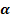# Dot Product calculator

This dot product calculator comes in handy when you have to solve vector multiplication problems that are not so easy to solve manually.

#### Dot Product Calculator

$$Vector \ A$$
$$Vector\ B$$

#### $$Input$$

$$(1,2,3)$$ $$(4,5,6)$$

#### Solution

$$\left(1, 2, 3\right)\cdot\left(4, 5, 6\right)=4 + 10 + 18 = 32$$

#### $$Dot\ Product\ Farmula$$

$$D=$$ $$i*j=|i|*|j|$$

## What is Dot Product calculator?

This dot product calculator comes in handy when you have to solve vector multiplication problems that are not so easy to solve manually. Rather than calculate your problems and scalar product by hand you can calculate the same problem with our tool which is really great and it’s doesn’t take much time and also you don’t have to solve it manually.

You just have to input the value of all the vectors and then you are ready to go and solve it. After you will enter the value in each section you don’t have to do anything else. You will see the result of all the problems you are looking for.

You have to read more and more to understand the formula and the tool so it will be easy for you to calculate the dot product and it will be easy to understand. Our tools have already set the formula by the developer to solve any problems regarding vector and Dot product calculation.

You have to learn how to calculate the dot product of two vectors and also you have to understand how to generalize the formula of metrics and that’s a good thing to read and learn about. While you start reading about this stuff then you will no longer face problems regarding algebra and vectors algebra isn’t anything to worry about.

## Types of vectors multiplications

There are two types of vector multiplications and those are the dot product calculator which (we also call the scalar product) and the dot product is mentioned by “.” and the Second one is cross-product that is represented by x. The difference between both products is that the Dot products which have only a single number while you do the operation, while the outcome of the cross product operation is vectors.

### Now let’s understand the dot product formula

Let’s suppose that all the calculations will be done in 3D space. That means every vector will be written down in 3 components as we have shown below.

a= [a1, a2, a3,] b= [b1, b2, b3]

The dot product is described as a vector product based on geometric and the magnitude multiplied by the cosine of the angle between them. This is how it can be expressed in equations.

a.b = |a| * |b| cos αAnd if you are not sure what is the magnitude of the vector is and even you don’t know how to calculate it then this tool is really good for you and if you want more details then you should check out our unit vector calculator.

You must have seen the angle between two vectors that is 90°, and then the scalar product is always will be zero 0, regardless of the magnitude of the vector. Similarly, if the angle is adequate to 0° (the vectors are collinear), then the scalar product is found by multiplying the multitudes only. In other words, the larger the relative slope between two vectors, the upper the worth of the scalar product.

Algebraically, the scalar product is the sum of products of the vectors' components. For three-component vectors, the scalar product formula looks as follows:

a·b = a₁ * b₁ + a₂ * b₂ + a₃ * b₃

In a space that has quite three dimensions, you merely got to add more terms to the summation. If on the opposite hand, you would like to multiply vectors during a 2D space, you've got to omit the third term of the formula.

The inner product calculator also can work as a tool to seek out the angle between two vectors that cosine is that the ratio between the scalar product and therefore the vectors' magnitudes:

Cos α = a·b / (|a| * |b|).

How to use this dot product calculator.

You must be wondering how our vector product is working? You just have to follow these steps to understand this tool how works.

• First choose your vectors value a. for an example a = [4, 5, -3] as in a= [a1, a2, a3].
• Choose your vector  b as in b=[b1, b2, b3] is b=[1, -2, -2]
• Now you have to calculate the product of the first components of each vector. And this is equal to 4*1=4.
• Calculate the merchandise of the second (middle) component of every vector. During this case, it's adequate to 5 * (-2) = -10.
• Calculate the merchandise of the third component of every vector. During this case, it's adequate to (-3) * (-2) = 6.
• Add all of those results together to seek out the scalar product of the vectors a and b. 4 + (-10) + 6 = 0.
• The results will be 0. This is often the inner product of those two vectors. It means they're perpendicular to every other (the angle between them is adequate to 90°).

A.